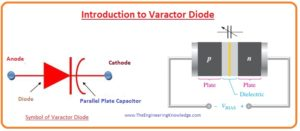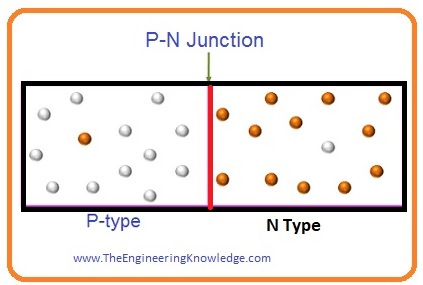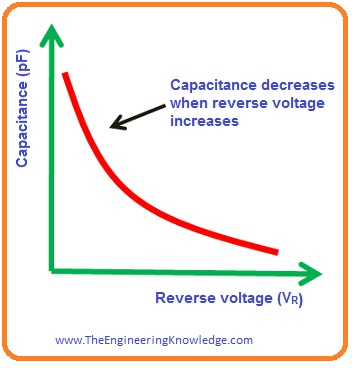Hello fellows, I hope you are doing great. In today’s tutorial, we will have a look at Introduction to Varactor Diode. The diode is an electronic component that has 2 terminals positive terminal and negative terminal positive terminal called an anode and negative called cathode. A diode is used for conversion of AC into DC, for this conversion rectifier circuits are used that uses diode. Rectifiers are further divided into two parts half-wave rectifiers and full-wave rectifiers.

There are numerous types of diode such as Zener diode, PIN diode, Schottky diode, varactor diode. In today’s post, we will have a detailed look at varactor diode its working, construction, applications, and some other related parameter. So let’s get started with Introduction to Varactor Diode.

#### Introduction to Varactor Diode

• The varactor diode is also known as variable capacitance diode, variable reactance diode, tuning diode.
• As we know Zener diode can operate in both reverse biased and forward biasing condition but the varactor diode always function in reverse biasing condition.
• Its doping level is high to increases capacitance of its depletion region, here the capacitance term is used because its operation is similar to the capacitor. We will discuss this behavior complete in coming lines.
• Similar to the dielectric material in the capacitor depletion region of varactor diode behaves like dielectric material.
• The P and N sides of the varactor capacitor function like the conductive plates of the capacitor. It is shown in the below figure.
• The symbol of varactor diode is also shown in the resultant figure.
• You can see that the symbol of this diode is alike to a normal diode.
• This diode also has anode and cathode like PN junction diode.
• One end is an anode and the second has 2 lines that represent the plates of a capacitor.
• The space between them represents their dielectric.
• This diode is also named as voltage-dependent diode it so called because its output relies on the value of the voltage at its input terminals.
• In such an application where different values of capacitance are needed varactor diode is used and its capacitance varies with the input voltage.#### Varactor Diode Construction

• For the construction of varactor diode P and N semiconductor materials are used.
• As we know that in N-type materials free electrons are majority charge carriers and holes are minority charge carriers.
• Due to the majority of electrons in N materials current flows.
• In P-type materials, current flows due to the holes as holes are majority carriers in P-type semiconductors.• A combination of P semiconductors With the N semiconductors PN junction is created among them.
• This PN junction is the partition between P and N semiconductors.
• In the depletion region, the positive and negative ions are exits that are linked with each other through the electric field.#### Working of Varactor Diode

• As we discuss varactor diode operates in reverse biasing condition. As we know in reverse biasing condition current movement is almost zero.
• If we apply voltage in forward biasing condition then current will pass through a diode.
• In forward biasing conditions, the area of the depletion region is very thin and ions in the depletion region are very less in thin region.
• If we apply a voltage across a diode in reverse biased condition the electrons in N part of diode moves towards the positive terminal of battery and holes in P part of diode move toward the negative point of battery.
• Due to the movement of electrons and holes the area of depletion region increases.
• As the varactor diode is similar to a capacitor due to the large size of depletion region capacitance will decrease according to the below equation.

C = AP∈/d• Though, if the provided reverse-biased voltage is less the capacitance will be very huge.
• The capacitance is in inverse relation to the width of the depletion region and direct proportion to the areas of N and P portions.
• The capacitance is in inverse relation to the width of the depletion region and direct proportion to the areas of N and P portions.
• If we increase the reverse-biased voltage the area of depletion region will increase and capacitance will more reduce.
• If we decrease reverse-biased voltage the area of the depletion region will reduce and capacitance will increases.• So an increment in reverse-biased voltage enhances the area of the depletion region and reduces the capacitance of varactor diode.
• The decrement in capacitance indicates that charge storage decreases.
• So the capacitance of diode can be regulated through the voltage variation.
• As we know in fixed capacitor there is no variation in the value of capacitance.
• While capacitance of varactor diode is varied with the voltage variation hence prove varactor is diode is a variable capacitor.
• The unit for the capacitance of the varactor diode is pF.

#### Varactor Diode in Tuning Circuit

• The most important use of varactor diode is tuning circuits. For instance VHF (Very high frequency), UHF (Ultra High frequency), and satellite receivers.
• Varactor diode is used in a mobile network system. When varactor diode is connected with the resonant circuitry as shown in the below figure it operates like a variable capacitor.• Using this circuitry we can set the frequency through varying the voltage.
• This diode delivers the net variable capacitance in parallel resonant bandpass filter.
• The combination of varactor diode and inductor creates a parallel resonant circuitry to the output terminals to AC ground.
• The capacitors in circuitry C1 and C2 have no influence on the frequency response of filter since their reactances are very less at the resonant frequency.
• The capacitor C1 stops a DC rout from the potentiometer wiper back to the alternating current (AC) supply through inductor and resistance R1.
• The capacitor C2 stops a dc rout from the potentiometer’s wiper to the load on the output.
• The potentiometer R2 generates a changeable dc voltage to provide biasing to the varactor diode.
• The formula for parallel resonant frequency is given below.

fr =1/2Π√LC

#### Varactor Diode Datasheet

• In below figure datasheet for Zetex 830 series is shown. Let’s discuss all parameters with the detailed.Capacitance Tolerance Range

• In the datasheet, you can see that minimum, nominal and maximum value of capacitance is shown.
• For instance, for reverse-biased three volts the current value of 832 ampere shows a capacitance among 19.8 PicoFarad to 24.2 PicoFarad.

Capacitance Ratio

• The capacitance ratio of varactor diode also called the tuning ratio. This ratio among the lowest reverse-biased voltage capacitance to extreme reverses biased voltage.
• In the above figure varactor diode represented has a capacitance ratio of the ratio between capacitance C calculated at a reverse-biased voltage (VR) of two volts divided by the capacitance calculated at reverse voltage VR of 20 volts.
• In this case capacitance ratio denoted as C2/C20.
• For 832-ampere current extreme capacitance ration is five volts.
• It shows that capacitance value reduces with the factor of five volts as VR increases from two volts to twenty volts.
• The given calculations explain how we can use capacitance ratio CR to calculate capacitance range of 832 amperes.

If C2=22 pF and lowest CR= C2/C20= 5

C20=C2/CR=22pF/5=4.4pF

• The capacitance of varactor diode changes from 22 picofarads to 4.4 picofarads when VR is enhanced from two volts to twenty volts.
• The varactor diode Zetex 830 series is hyper–abrupt junction modules. The doping in N and P parts is designed uniformly so at PN junction there is a sudden variation from N to P while in diode used for rectifiers variation is slow.
• The capacitance ration finds by the abruptness of the PN junction.

Varactor Diode Back-to-Back Configuration

• There is one disadvantage if we use one varactor diode in circuitry such as radio frequency then during forward biasing through the radio signal frequency leakage current will increase for a short time interval.
• The harmonic distortion is created if a diode is consecutively biased positively and negatively.
• For the reduction of harmonic distortion in the figure you can see 2 diodes are connected in back to back configuration.• Across these diodes, a reverse-biased dc voltage is provided at the same time.
• These 2 diodes will have high and less value of capacitance in alternative way total capacitance will have constant value and will not affect through the radio frequency amplitude.
• The Zetex 832 ampere rating diode is exited in back to back configuration in the packaging of SOT23 shown in the below figure.• Cathode terminals of back to the back configuration are attached to common pinout instead of that every diode in this configuration can also be used lonely.

#### Related Posts

1. Fast Private Proxies says:

Have you ever thought about including a little bit more than just your articles? I mean, what you say is important and all. But think about if you added some great visuals or videos to give your posts more, “pop”! Your content is excellent but with images and video clips, this blog could certainly be one of the most beneficial in its field. Superb blog!

2. Alison Imel says:

I have been surfing online more than 2 hours today, yet I never found any interesting article like yours. It is pretty worth enough for me. In my view, if all web owners and bloggers made good content as you did, the web will be a lot more useful than ever before.

3. Lesha Maler says:

Wow, incredible blog layout! How long have you been blogging for? you make blogging look easy. The overall look of your site is wonderful, as well as the content!

4. ปั้มไลค์ says:

Like!! I blog quite often and I genuinely thank you for your information. The article has truly peaked my interest.

5. best web hosting sites says:

Hello! This post could not be written any better!
Reading this post reminds me of my previous room mate!